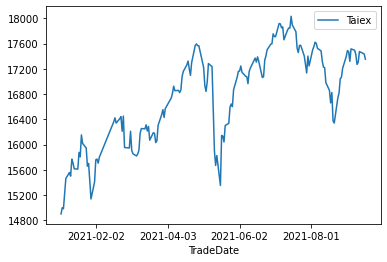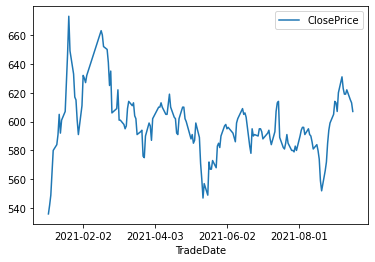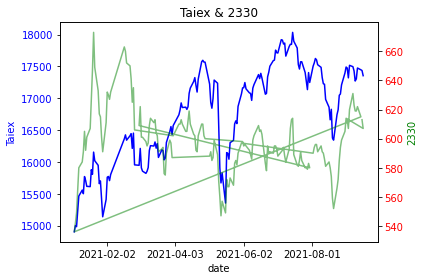DAY 15
0
Software Development

取得資料

加權指數今年的收盤點位

# 加權指數畫圖
ax = plt.gca()
# 設定Ｘ軸刻度
ax.xaxis.set_major_locator(matplotlib.ticker.MaxNLocator(5))
# 設定y軸刻度
ax.yaxis.set_major_locator(matplotlib.ticker.MaxNLocator(10))
plt.plot()台積電的今年收盤價

df_2330 = pandas.read_sql("SELECT * FROM DailyPrice WHERE Symbol ='2330' and TradeDate > '2021-01-01';", con=conn)

# 台積電畫圖
ax = plt.gca()
# 設定Ｘ軸刻度
ax.xaxis.set_major_locator(matplotlib.ticker.MaxNLocator(5))
# 設定y軸刻度
ax.yaxis.set_major_locator(matplotlib.ticker.MaxNLocator(10))
plt.plot()比較大盤與台積電關係

# 畫圖
# 在畫圖的地方，無法寫中文的名稱
fig, ax1 = plt.subplots()
plt.title("Taiex & 2330")
plt.xlabel("date")
ax2 = ax1.twinx()

# 製作第一個圖表：折線圖
# blue：表示藍色，可以用「b」表示
ax1.set_ylabel("Taiex", color="blue")
ax1.tick_params(axis="y", labelcolor="blue")

# 製作第二個圖表：折線圖
ax2.set_ylabel("2330", color="green")
ax2.plot(df_2330["TradeDate"], df_2330["ClosePrice"], color="green", alpha=0.5) # alpha: 增加透明度
ax2.tick_params(axis="y", labelcolor="r")

# 設定Ｘ軸刻度
ax = plt.gca()
ax.xaxis.set_major_locator(matplotlib.ticker.MaxNLocator(5))
# 設定y軸刻度
ax.yaxis.set_major_locator(matplotlib.ticker.MaxNLocator(10))

fig.tight_layout()
plt.show()